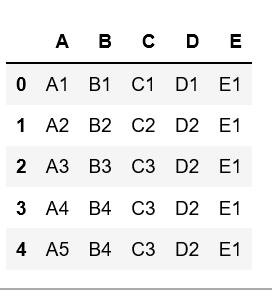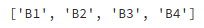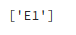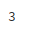# Get unique values from a column in Pandas DataFrame

Let’s discuss how to get unique values from a column in Pandas DataFrame.

Create a simple dataframe with dictionary of lists, say columns name are A, B, C, D, E with duplicate elements.Now, let’s get the unique values of a column in this dataframe.

Example #1: Get the unique values of ‘B’ column

 `# Import pandas package  ` `import` `pandas as pd ` ` `  `# create a dictionary with five fields each ` `data ``=` `{ ` `    ``'A'``:[``'A1'``, ``'A2'``, ``'A3'``, ``'A4'``, ``'A5'``],  ` `    ``'B'``:[``'B1'``, ``'B2'``, ``'B3'``, ``'B4'``, ``'B4'``],  ` `    ``'C'``:[``'C1'``, ``'C2'``, ``'C3'``, ``'C3'``, ``'C3'``],  ` `    ``'D'``:[``'D1'``, ``'D2'``, ``'D2'``, ``'D2'``, ``'D2'``],  ` `    ``'E'``:[``'E1'``, ``'E1'``, ``'E1'``, ``'E1'``, ``'E1'``] } ` ` `  `# Convert the dictionary into DataFrame  ` `df ``=` `pd.DataFrame(data) ` ` `  `# Get the unique values of 'B' column ` `df.B.unique() `

Output:Example #2: Get the unique values of ‘E’ column

 `# Import pandas package  ` `import` `pandas as pd ` ` `  `# create a dictionary with five fields each ` `data ``=` `{ ` `    ``'A'``:[``'A1'``, ``'A2'``, ``'A3'``, ``'A4'``, ``'A5'``],  ` `    ``'B'``:[``'B1'``, ``'B2'``, ``'B3'``, ``'B4'``, ``'B4'``],  ` `    ``'C'``:[``'C1'``, ``'C2'``, ``'C3'``, ``'C3'``, ``'C3'``],  ` `    ``'D'``:[``'D1'``, ``'D2'``, ``'D2'``, ``'D2'``, ``'D2'``],  ` `    ``'E'``:[``'E1'``, ``'E1'``, ``'E1'``, ``'E1'``, ``'E1'``] } ` ` `  `# Convert the dictionary into DataFrame  ` `df ``=` `pd.DataFrame(data) ` ` `  `# Get the unique values of 'E' column ` `df.E.unique() `

Output:Example #3: Get number of unique values in a column

 `# Import pandas package  ` `import` `pandas as pd ` ` `  `# create a dictionary with five fields each ` `data ``=` `{ ` `    ``'A'``:[``'A1'``, ``'A2'``, ``'A3'``, ``'A4'``, ``'A5'``],  ` `    ``'B'``:[``'B1'``, ``'B2'``, ``'B3'``, ``'B4'``, ``'B4'``],  ` `    ``'C'``:[``'C1'``, ``'C2'``, ``'C3'``, ``'C3'``, ``'C3'``],  ` `    ``'D'``:[``'D1'``, ``'D2'``, ``'D2'``, ``'D2'``, ``'D2'``],  ` `    ``'E'``:[``'E1'``, ``'E1'``, ``'E1'``, ``'E1'``, ``'E1'``] } ` ` `  `# Convert the dictionary into DataFrame  ` `df ``=` `pd.DataFrame(data) ` ` `  `# Get number of unique values in column 'C' ` `df.C.nunique(dropna ``=` `True``) `

Output:Attention geek! Strengthen your foundations with the Python Programming Foundation Course and learn the basics.

To begin with, your interview preparations Enhance your Data Structures concepts with the Python DS Course.

My Personal Notes arrow_drop_upStrategy Path planning and Destination matters in success No need to worry about in between temporary failures

If you like GeeksforGeeks and would like to contribute, you can also write an article using contribute.geeksforgeeks.org or mail your article to contribute@geeksforgeeks.org. See your article appearing on the GeeksforGeeks main page and help other Geeks.

Please Improve this article if you find anything incorrect by clicking on the "Improve Article" button below.

Article Tags :

1

Please write to us at contribute@geeksforgeeks.org to report any issue with the above content.